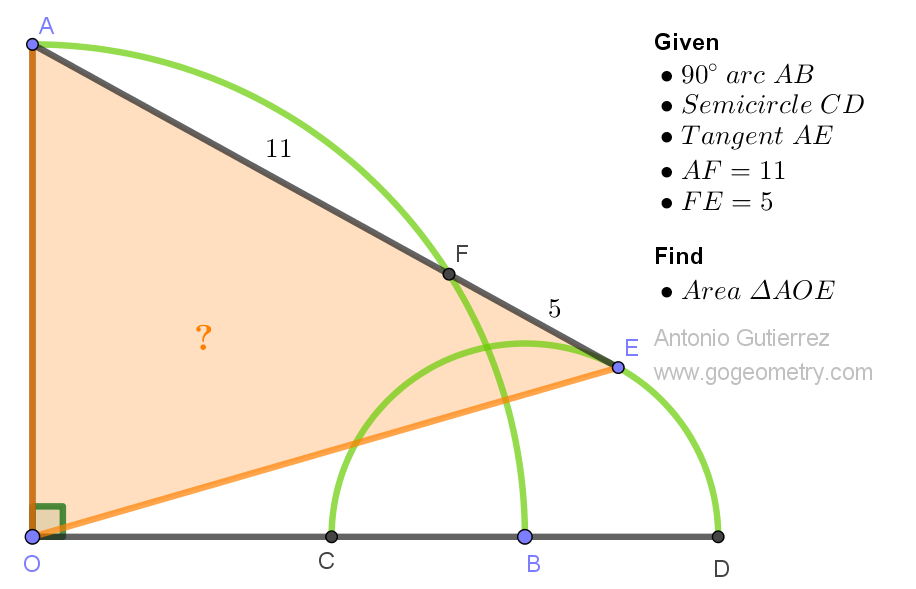# Geometry Problem 1544 Challenge: Calculate the Area of a Triangle with Given Arc and Semicircle Intersections

Given the 90-degree arc AB with center at O and the semicircle with center at B and diameter CD (where C lies on OB and D lies on the extension of OB), draw the tangent AE from A to the semicircle, intersecting the arc AB at F (with E as the point of tangency). If AF measures 11 and FE measures 5, calculate the area of triangle AOE.Geometry's riddle,
Problem 1544 challenge,
Area to unveil.
Arc and semicircle meet,
Triangle's secrets revealed.

Challenge yourself with this captivating problem and unlock the beauty of geometric calculations. Explore the intricate connections between arcs, semicircles, and triangles.